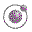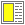Higher-order Functions - slide 17 : 34

Accumulation

It is not satisfactory that we cannot reduce the empty list

We remedy the problem by passing an extra parameter to the reduction functions

We call this variant of the reduction functions for accumulationThe function accumulate-right.
 Expression Value `(accumulate-right - 0 '())` `0` `(accumulate-right - 0 '(1 2 3 4 5))` `3` ```(accumulate-right append '() (list (list 1 2 3) (list 'a 'b 'c)))``` `(1 2 3 a b c)`
In relation to web programming we most often append accumulate lists and strings

accumulate-right is part of the general LAML library

Due to their deficiencies, the reduction functions are not used in LAML